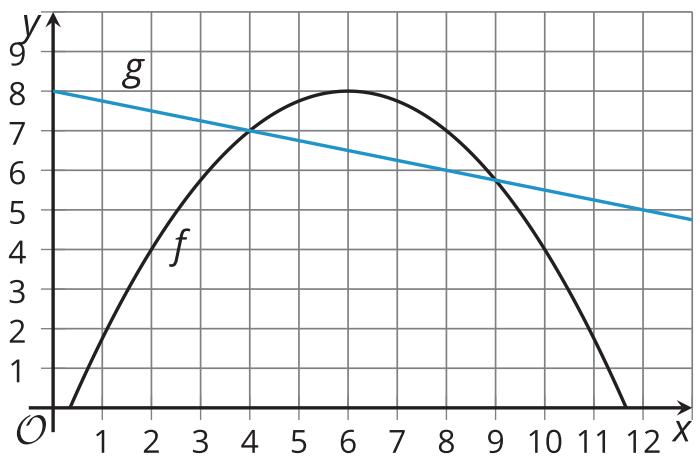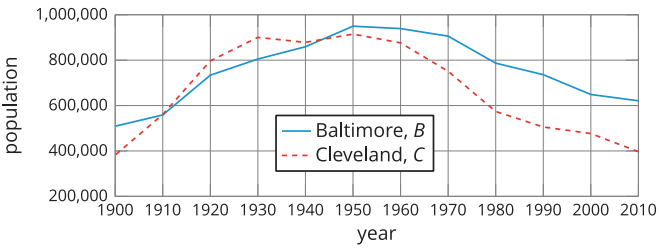# A.4.9.4 Functions f and g

Topic:
Functions

Here are graphs that represent two functions, f and g. Decide which function value is greater for each given input. Be prepared to explain your reasoning.1. a. f(2) or g(2) b. f(4) or g(4) c. f(6) or g(6) d. f(8) or g(8) 2. Is there a value of x at which the equation f(x)=g(x) is true? Explain your reasoning. 3. Identify at least two values of x at which the inequality f(x)

Here is the population graphs for Baltimore and Cleveland from 1900 to 2010, which we looked at in the opener.Below are 5 statements about the populations of the two cities. Explain how you could tell from the graphs that each statement is true, and translate each verbal description into a statement with the same meaning but written in function notation. The first one is done for you. Statement #1: In 2010, Baltimore had more people than Cleveland. Answer: The graph of C is below the graph of B when x is equal to 2010 C(2010) < B(2010)

Statement #2: Baltimore and Cleveland had the same population twice in the past century, in 1910 and around 1944.

Statement #3: After the mid-1940's, Cleveland has a smaller population than Baltimore.

Statement #4: In the first half of the 20th century, the population of Cleveland grew faster than that of Baltimore.

Statement #5: Since 1950, the population of Cleveland has dropped at a faster rate than that of Baltimore.